# Align text to nearest line.## Recommended PostsMy boss may have just asked for the impossible, but he wants a lisp to be able to window select multiple text then window select lines near the text, and have the text align to the line or polyline that is closest to the text. I have something that allows me to pick a line then a text, but I have no idea how to do this. Any help is greatly appreciated, this is way above my ability. The attached pictures show before and after.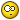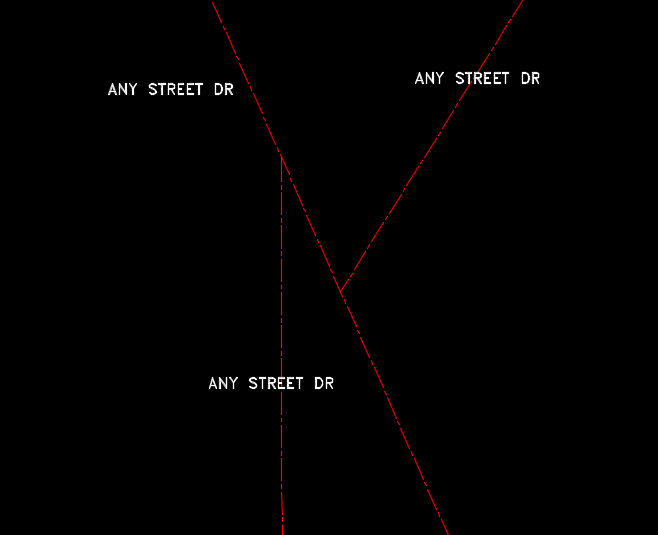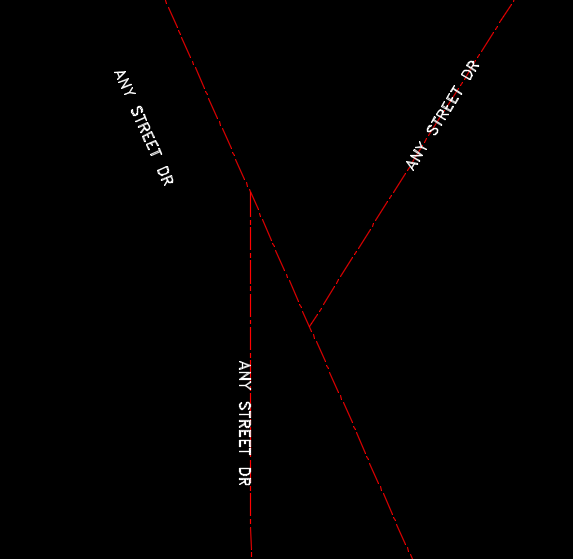• Replies 53
• Created

#### Top Posters In This Topic

•18

•7

•6

•4

#### Top Posters In This Topic

•ronjonp 18 posts

•rog1n 7 posts

•Mitch Leach 6 posts

•aawilds 4 posts

#### Popular PostsThis is probably what you need.   http://www.lee-mac.com/curvealignedtext.htmlThe better way is to draw the pline and label at that time. If its lengths etc. label inside outside. I don't have anything. This is a built in function of CIV3D.Is it possible to get a thank you first ? 🤨

#### Posted ImagesThis is probably what you need.

http://www.lee-mac.com/curvealignedtext.html

•1
##### Share on other sitesI had looked at that Lisp by Lee mac. It is good, but I need to be able to select multiple text that are already in the drawing, and them be aligned to the nearest line. Without having to pick individually.

##### Share on other sitesI had looked at that Lisp by Lee mac. It is good, but I need to be able to select multiple text that are already in the drawing, and them be aligned to the nearest line. Without having to pick individually.

Give this a try:

```(defun c:foo (/ a l lines p ss text)
(if (setq ss (ssget '((0 . "Line,Text"))))
(progn (foreach x (vl-remove-if 'listp (mapcar 'cadr (ssnamex ss)))
(if (= "TEXT" (cdr (assoc 0 (entget x))))
(setq text (cons x text))
(setq lines (cons x lines))
)
)
(if lines
(foreach x	text
(setq p (cdr (assoc 10 (entget x))))
(setq l (car (vl-sort lines
'(lambda (a b)
(< (distance p (vlax-curve-getclosestpointto a p))
(distance p (vlax-curve-getclosestpointto b p))
)
)
)
)
)
(setq a (angle (cdr (assoc 10 (entget l))) (cdr (assoc 11 (entget l)))))
(entmod (subst (cons 50 a) (assoc 50 (entget x)) (entget x)))
)
)
)
)
(princ)
)```

##### Share on other sitesThat works for the most part. Is there any way to get it to work with polylines?

##### Share on other sitesThe answer for plines is you can get closest point to and then do a "first derivative" which will allow you to work out an angle for the text. look at this bit of code.

```(defun alg-ang (obj pnt)
(angle '(0. 0. 0.)
(vlax-curve-getfirstderiv
obj
(vlax-curve-getparamatpoint
obj
pnt
)
)
)
)
```

##### Share on other sitesThat works for the most part. Is there any way to get it to work with polylines?

It's very easy to include polylines, but your initial question only asked for lines and text. With the code provided, just explode polylines and it should work per the initial request.

##### Share on other sitesThank You ronjonp and BIGAL. You both have been very helpful.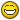##### Share on other sitesHere's a version that will work with polylines too:

```(defun c:foo (/ _aap l lines p p2 ss text)
;; RJP - 6.14.2017
(defun _aap (ename pt / param)
(if	(and (not (vl-catch-all-error-p (vl-catch-all-apply 'vlax-curve-getendparam (list ename))))
(setq param (vlax-curve-getparamatpoint ename pt))
)
(angle '(0 0) (vlax-curve-getfirstderiv ename param))
)
)
(if (setq ss (ssget '((0 . "*polyline,Line,Text"))))
(progn (foreach x (vl-remove-if 'listp (mapcar 'cadr (ssnamex ss)))
(if (= "TEXT" (cdr (assoc 0 (entget x))))
(setq text (cons x text))
(setq lines (cons x lines))
)
)
(if lines
(foreach x	text
(setq p (cdr (assoc 10 (entget x))))
(setq
l (mapcar
'(lambda (x)
(list (setq p2 (vlax-curve-getclosestpointto x p)) (distance p p2) (_aap x p2))
)
lines
)
)
(setq l (car (vl-sort l '(lambda (a b) (< (cadr a) (cadr b))))))
(entmod (subst (cons 50 (caddr l)) (assoc 50 (entget x)) (entget x)))
)
)
)
)
(princ)
)```

##### Share on other sitesThat's really good Ron thanks. I was wondering how to make the text readable? I cant see how to simply integrate Alans routine in to yours http://cadtips.cadalyst.com/multiline-text/make-text-readable

any ideas?

Thanks P

##### Share on other sitesThat's really good Ron thanks. I was wondering how to make the text readable? I cant see how to simply integrate Alans routine in to yours http://cadtips.cadalyst.com/multiline-text/make-text-readable

any ideas?

Thanks P

There are a ton of makereadable functions HERE. Just apply one of them to this part of the line here in red: (entmod (subst (cons 50 (caddr l)) (assoc 50 (entget x)) (entget x)))

##### Share on other sites
• 2 years later...On 6/14/2017 at 2:56 AM, ronjonp said:

Give this a try:

```
(defun c:foo (/ a l lines p ss text)
(if (setq ss (ssget '((0 . "Line,Text"))))
(progn (foreach x (vl-remove-if 'listp (mapcar 'cadr (ssnamex ss)))
(if (= "TEXT" (cdr (assoc 0 (entget x))))
(setq text (cons x text))
(setq lines (cons x lines))
)
)
(if lines
(foreach x	text
(setq p (cdr (assoc 10 (entget x))))
(setq l (car (vl-sort lines
'(lambda (a b)
(< (distance p (vlax-curve-getclosestpointto a p))
(distance p (vlax-curve-getclosestpointto b p))
)
)
)
)
)
(setq a (angle (cdr (assoc 10 (entget l))) (cdr (assoc 11 (entget l)))))
(entmod (subst (cons 50 a) (assoc 50 (entget x)) (entget x)))
)
)
)
)
(princ)
)```

i found this

just what i need. but can someone add alingment of the text to the midpoint of the line?

all the text are in justify middle center. and i need the texts to be in midpoints of the lines

i mean my texts are in above and some are below the line, i want the text alinged as well to the mid point of the line

Edited by ktbjx
##### Share on other sitesGive this a try:

```(defun c:foo (/ a l lines mp p p1 p2 ss text)
(if (setq ss (ssget ":L" '((0 . "Line,Text"))))
(progn (foreach x (vl-remove-if 'listp (mapcar 'cadr (ssnamex ss)))
(if (= "TEXT" (cdr (assoc 0 (entget x))))
(setq text (cons x text))
(setq lines (cons x lines))
)
)
(if lines
(foreach x	text
(setq p (cdr (assoc 10 (entget x))))
(setq l (car (vl-sort lines
'(lambda (a b)
(< (distance p (vlax-curve-getclosestpointto a p))
(distance p (vlax-curve-getclosestpointto b p))
)
)
)
)
)
(setq p1 (cdr (assoc 10 (entget l))))
(setq p2 (cdr (assoc 11 (entget l))))
(setq mp (polar p1 (setq a (angle p1 p2)) (/ (distance p1 p2) 2)))
(entmod (subst (cons 50 a) (assoc 50 (entget x)) (entget x)))
(entmod (subst (cons 10 mp) (assoc 10 (entget x)) (entget x)))
(entmod (subst (cons 11 mp) (assoc 11 (entget x)) (entget x)))
)
)
)
)
(princ)
)```

##### Share on other sites9 hours ago, ronjonp said:

Give this a try:

```
(defun c:foo (/ a l lines mp p p1 p2 ss text)
(if (setq ss (ssget ":L" '((0 . "Line,Text"))))
(progn (foreach x (vl-remove-if 'listp (mapcar 'cadr (ssnamex ss)))
(if (= "TEXT" (cdr (assoc 0 (entget x))))
(setq text (cons x text))
(setq lines (cons x lines))
)
)
(if lines
(foreach x	text
(setq p (cdr (assoc 10 (entget x))))
(setq l (car (vl-sort lines
'(lambda (a b)
(< (distance p (vlax-curve-getclosestpointto a p))
(distance p (vlax-curve-getclosestpointto b p))
)
)
)
)
)
(setq p1 (cdr (assoc 10 (entget l))))
(setq p2 (cdr (assoc 11 (entget l))))
(setq mp (polar p1 (setq a (angle p1 p2)) (/ (distance p1 p2) 2)))
(entmod (subst (cons 50 a) (assoc 50 (entget x)) (entget x)))
(entmod (subst (cons 10 mp) (assoc 10 (entget x)) (entget x)))
(entmod (subst (cons 11 mp) (assoc 11 (entget x)) (entget x)))
)
)
)
)
(princ)
)```

Sir, the text went do the midpoint of the line...

i just want the center point of the text to be aligned with the midpoint of the line. like this:

##### Share on other sitesTry this. In the future post a drawing with your question first so the translation does not get lost.```(defun c:foo (/ a h l lines mp p p1 p2 p3 p4 pa s text)
;; RJP » 2019-08-07
(if (setq s (ssget ":L" '((0 . "Line,Text"))))
(progn (foreach x (vl-remove-if 'listp (mapcar 'cadr (ssnamex s)))
(if (= "TEXT" (cdr (assoc 0 (entget x))))
(setq text (cons x text))
(setq lines (cons x lines))
)
)
(if lines
(foreach x	text
(setq p (cdr (assoc 11 (entget x))))
(setq l (car (vl-sort lines
'(lambda (a b)
(< (distance p (vlax-curve-getclosestpointto a p))
(distance p (vlax-curve-getclosestpointto b p))
)
)
)
)
)
(setq h (* 0.75 (cdr (assoc 40 (entget x)))))
(setq p1 (cdr (assoc 10 (entget l))))
(setq p2 (cdr (assoc 11 (entget l))))
(setq mp (polar p1 (setq a (angle p1 p2)) (/ (distance p1 p2) 2)))
(setq p3 (polar mp (setq pa (+ (/ pi 2) a)) h))
(setq p4 (polar mp (+ pi pa) h))
(if (< (distance p p4) (distance p p3))
(setq p3 p4)
)
(entmod (subst (cons 50 a) (assoc 50 (entget x)) (entget x)))
(entmod (subst (cons 10 p3) (assoc 10 (entget x)) (entget x)))
(entmod (subst (cons 11 p3) (assoc 11 (entget x)) (entget x)))
)
)
)
)
(princ)
)```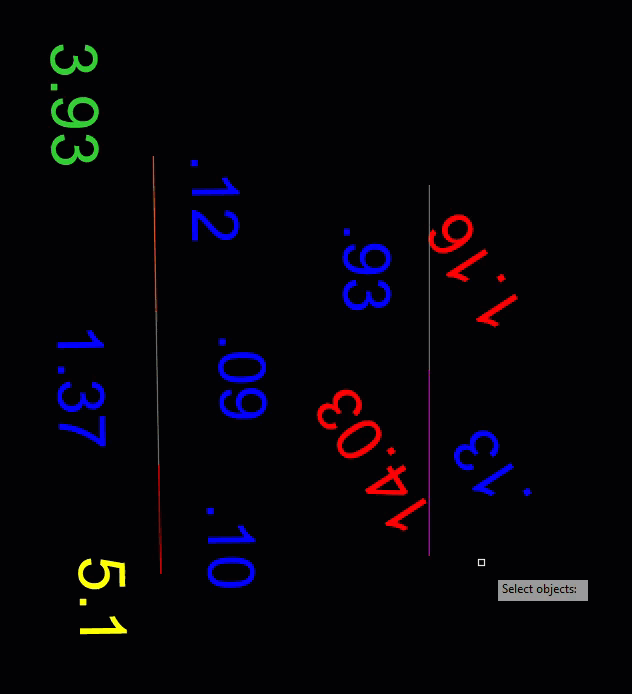##### Share on other sitesHello, Ranjani if it's not too much to ask, is it possible to modify the lisp to work with closed polylines and the text aligned with the middle lines inside of the formed draw?##### Share on other sitesThe better way is to draw the pline and label at that time. If its lengths etc. label inside outside. I don't have anything. This is a built in function of CIV3D.

•1
##### Share on other sitesOn 8/7/2019 at 11:20 AM, ronjonp said:

Try this. In the future post a drawing with your question first so the translation does not get lost.```
(defun c:foo (/ a h l lines mp p p1 p2 p3 p4 pa s text)
;; RJP » 2019-08-07
(if (setq s (ssget ":L" '((0 . "Line,Text"))))
(progn (foreach x (vl-remove-if 'listp (mapcar 'cadr (ssnamex s)))
(if (= "TEXT" (cdr (assoc 0 (entget x))))
(setq text (cons x text))
(setq lines (cons x lines))
)
)
(if lines
(foreach x	text
(setq p (cdr (assoc 11 (entget x))))
(setq l (car (vl-sort lines
'(lambda (a b)
(< (distance p (vlax-curve-getclosestpointto a p))
(distance p (vlax-curve-getclosestpointto b p))
)
)
)
)
)
(setq h (* 0.75 (cdr (assoc 40 (entget x)))))
(setq p1 (cdr (assoc 10 (entget l))))
(setq p2 (cdr (assoc 11 (entget l))))
(setq mp (polar p1 (setq a (angle p1 p2)) (/ (distance p1 p2) 2)))
(setq p3 (polar mp (setq pa (+ (/ pi 2) a)) h))
(setq p4 (polar mp (+ pi pa) h))
(if (< (distance p p4) (distance p p3))
(setq p3 p4)
)
(entmod (subst (cons 50 a) (assoc 50 (entget x)) (entget x)))
(entmod (subst (cons 10 p3) (assoc 10 (entget x)) (entget x)))
(entmod (subst (cons 11 p3) (assoc 11 (entget x)) (entget x)))
)
)
)
)
(princ)
)```

Is possible change to work with polyline too?

##### Share on other sites3 minutes ago, rog1n said:

Is possible change to work with polyline too?

Is it possible to get a thank you first ?Edited by ronjonp
•1
##### Share on other sites2 minutes ago, ronjonp said:

Is it possible to get a thank you first ?sorry I had never noticed this option## Join the conversation

You can post now and register later. If you have an account, sign in now to post with your account.
Note: Your post will require moderator approval before it will be visible.×   Pasted as rich text.   Restore formatting

Only 75 emoji are allowed.